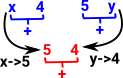# Unification

### Syntactic Unification

The aim is to make terms syntactically equivalent by substituting constants for variables in the most general way.

Usually these variables and constants represent first order terms (arity=0), but there is also higher order unification where we might have variables representing functions.

This subject is about unifying expressions with terms containing constants and variables such as:

• f(x,4)
• f(5,y)

which might be unified to:

• f(5,4)

Unification is a generalisation of pattern matching where the constants and variables may be in either expression.

 These expressions are tree structures and we are substituting subtrees with other subtrees. The unification problem could also be formulated with a graph or other mathematical structure. So 'f' is a binary expression and 'x', 'y', '4' and '5' are unary expressionsHere is a classical unification algorithm to generate the most general unification (MGU) of two terms. ```unify : t:term -> s:term -> substitution unify t s = if t=s then return {} if (isVariable t) & (isVariable s) then return {t<-s} if (isVariable t) then if (aVariableIn t s) // is t a variable in term s then return fail // can't have cycle else return {t<-s} if (isVariable s) then if (aVariableIn s t) // is s a variable in term t then return fail // can't have cycle else return {s<-t} if (t.name = s.name) & (t.arity = s.arity) // function name and arity must match // constants are 0-arity functions then // generate substitution for each parameter r={} for a1 in t for a2 in s do r = concatinate r (unify a1 a2) // combine substitutions return r else return fail // function don't match```

### Equational Unification

Make terms equationally equivalent.

The expressions must be matched 'modulo E' where E is the equations (identities) that must hold.

 So we could take the previous example but this time use say '+' which has other properties. It is commutative (x+y)=(y+x) so this allows other solutions.